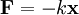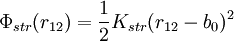Harmonic spring approximation${\mathbf F} = -k {\mathbf x}$$\Phi_{str} (r_{12}) = \frac{1}{2} K_{str} ( r_{12} - b_0 )^2$